# Plane Figures and Solid Shapes

## Definition

• Dimension: Dimensions in mathematics are the measure of the size or distance of an object or region or space in one direction. In simpler terms, it is the measurement of the length, width, and height of anything.
• Zero-dimensional: A point is a zero-dimensional object as it has no length, width, or height. It has no size. It tells about the location only. A point is dimensionless.
• One Dimensional: A line segment drawn on a surface is a one-dimensional object, as it has only length and no width.
• The 2-dimensional shapes or Plane Figures: The 2-dimensional shapes or objects in geometry are flat plane figures that have two dimensions – length and width.
• The 3-dimensional shapes or solid object: A solid object has three measurements like length, breadth, height, or depth. Hence, they are called three-dimensional shapes. Also, a solid object occupies some space

## Notes

### Plane Figures and Solid Shapes:

#### What are Dimensions?

Dimensions in mathematics are the measure of the size or distance of an object or region or space in one direction. In simpler terms, it is the measurement of the length, width, and height of anything.

In our day to day life, we see several objects like books, balls, ice-cream cones, etc., around us which have different shapes.

Any object or space can be

One-dimensional (or 1D)

Two-dimensional (or 2D)

Three-dimensional (or 3D)

For example,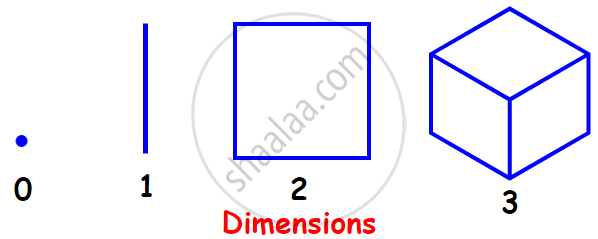#### 1. Zero Dimensional:

A point is a zero-dimensional object as it has no length, width, or height. It has no size. It tells about the location only. A point is dimensionless.#### 2. One Dimensional:

A line segment drawn on a surface is a one-dimensional object, as it has only length and no width.
For example,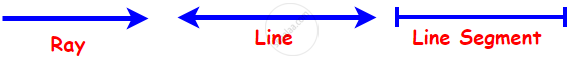#### 3. Two Dimensional:

The 2-dimensional shapes or objects in geometry are flat plane figures that have two dimensions – length and width. Two-dimensional or 2-D shapes do not have any thickness and can be measured in only two faces.

A plane figure can be made of straight lines, curved lines, or both straight and curved lines. The circle, the square, the rectangle, the quadrilateral, and the triangle are examples of plane figures.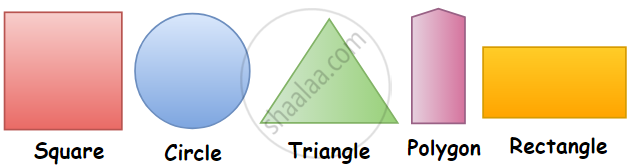#### 4. Three-dimensional shapes:

• A solid object has three measurements like length, breadth, height, or depth. Hence, they are called three-dimensional shapes. Also, a solid object occupies some space.

• The cube, the cuboid, the sphere, the cylinder, the cone, and the pyramid are examples of solid shapes.

• 3D objects have different views from different positions.

• We can measure their volume, Curved Surface Area, Lateral Surface Area, or Total Surface Area.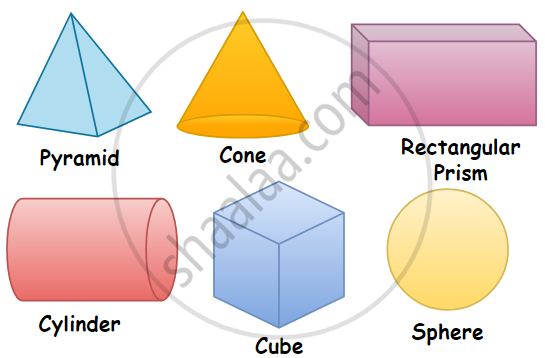### 3-dimensional shapes

2-dimensional shapes have only length and breadth.

3-dimensional shapes have length, breadth, height, or depth.

Plane figures are of two-dimensions (2-D).

Solid shapes are of three-dimensions (3-D).

The circle, the square, the rectangle, the quadrilateral and the triangle are examples of plane figures.

The cube, the cuboid, the sphere, the cylinder, the cone, and the pyramid are examples of solid shapes.

2D objects do not have different views from different positions.

3D objects have different views from different positions.

We can measure their area and Perimeter.

We can measure their volume, Curved Surface Area, Lateral Surface Area or Total Surface Area.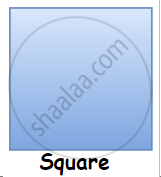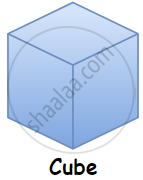If you would like to contribute notes or other learning material, please submit them using the button below.

### Shaalaa.com

3D and 2D Shapes [00:04:39]
S
0%